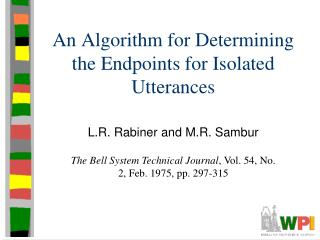DownloadDownload PresentationAn Algorithm for Determining the Endpoints for Isolated Utterances

# An Algorithm for Determining the Endpoints for Isolated Utterances

Télécharger la présentation## An Algorithm for Determining the Endpoints for Isolated Utterances

- - - - - - - - - - - - - - - - - - - - - - - - - - - E N D - - - - - - - - - - - - - - - - - - - - - - - - - - -
##### Presentation Transcript

1. An Algorithm for Determining the Endpoints for Isolated Utterances L.R. Rabiner and M.R. Sambur The Bell System Technical Journal, Vol. 54, No. 2, Feb. 1975, pp. 297-315

2. Outline • Intro to problem • Solution • Algorithm • Summary

3. Motivation • Word recognition needs to detect word boundaries in speech • Recognizing silence can reduce: • Processing load • (Network not identified as savings source) • Relatively easy in sound proof room, with digitized tape

4. Visual Recognition • Easy • Note how quiet beginning is (tape) “Eight”

5. Slightly Tougher Visual Recognition • “sss” starts crossing the ‘zero’ line, so can still detect “Six”

6. Tough Visual Recognition • Eye picks ‘B’, but ‘A’ is real start • /f/ is a weak fricative “Four”

7. Tough Visual Recognition • Eye picks ‘A’, but ‘B’ is real endpoint • V becomes devoiced “Five”

8. Tough Visual Recognition • Difficult to say where final trailing off ends “Nine”

9. The Problem • Noisy computer room with background noise • Weak fricatives: /f, th, h/ • Weak plosive bursts: /p, t, k/ • Final nasals (ex: “nine”) • Voiced fricatives becoming devoiced (ex: “five”) • Trailing off of sounds (ex: “binary”, “three”) • Simple, efficient processing • Avoid hardware costs

10. The Solution • Two measurements: • Energy • Zero crossing rate • Show: simple, fast, accurate

11. Energy • Sum of magnitudes of 10 ms of sound, centered on interval: • E(n) = i=-50 to 50 |s(n + i)|

12. Zero (Level) Crossing Rate • Number of zero crossings per 10 ms • Normal number of cross-overs during silence • Increase in cross-overs during speech

13. The Algorithm: Startup • At initialization, record sound for 100ms • Assume ‘silence’ • Measure background noise • Compute average (IZC’) and std dev () of zero crossing rate • Choose Zero-crossing threshold (IZCT) • Threshold for unvoiced speech • IZCT = min(25 / 10ms, IZC’ + 2 )

14. The Algorithm: Thresholds • Compute energy, E(n), for interval • Get max, IMX • Have silence, IMN I1 = 0.03 * (IMX – IMN) + IMN (3% of peak energy) I2 = 4 * IMN (4x silent energy) • Get energy thresholds (ITU and ITL) • ITL = MIN(I1, I2) • ITU = 5 * ITL

15. The Algorithm: Energy Computation • Search sample for energy greater than ITL • Save as start of speech, say s • Search for energy greater than ITU • s becomes start of speech • If energy falls below ITL, restart • Search for energy less than ITL • Save as end of speech • Results in conservative estimates • Endpoints may be outside

16. The Algorithm: Zero Crossing Computation • Search back 250 ms • Count number of intervals where rate exceeds IZCT • If 3+, set starting point, s, to first time • Else s remains the same • Do similar search after end

17. The Algorithm: Example (Word begins with strong fricative)

18. Algorithm: Examples • Caught trailing /f/ “Half”

19. Algorithm: Examples “Four” (Notice how different each “four” is)

20. Evaluation: Part 1 • 54-word vocabulary • Read by 2 males, 2 females • No gross errors (off by more than 50ms) • Some small errors • Losing weak fricatives • None affected recognition

21. Evaluation: Part 2 • 10 speakers • Count 0 to 9 • No errors at all

22. Evaluation: Part 3 • Your Project 1b…